# O modo

• Nota de Estudos
• Rever Tópicos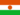Mounkaila D. 0 0 good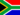No M. 0 0 Mode has the highest frequency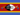Samkeliso R S. 0 0 highest frequency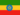Henock A. 0 0 done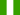Juliet N. 0 0 HIGHEST OCCURING SCORE IN A FREQUENCY DISTRIBUTION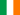Rachel N. 0 0 The mode is the most frequently occurring score.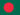Jahidul I. 0 0 The mode is the most frequently occurring score. The mode is the value that appears most often in a set of data. The mode of a discrete probability distribution is the value x at which its probability mass function takes its maximum value. In other words, it is the value that is most likely to be sampled. The mode of a continuous probability distribution is the value x at which its probability density function has its maximum value, so, informally speaking, the mode is at the peakJahidul I. 0 0 How to Make a Cumulative Frequency Table of Data ?
• Text Version### Você recebeu uma nova notificação

Clique aqui para visualizar todos eles